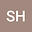Determination of the Eigenfunction's and Eigenvalue's of Quantum dots by FDTD
•• sedighe shanan hayavi
sedighe shanan hayavi
Shahid Chamran University of Ahvaz

Corresponding Author:[email protected]

Author Profile## Abstract

In this Article, we consider quantum dots with different structures and solve the Schrödinger equations for them by finite difference time-domain method and obtain the Eigenfunction’s and the Eigenvalue’s of these points.The FDTD method have an analysis that demonstrates the high accuracy of this method for solving quantum equations.The FDTD method is a suitable method for simulating electromagnetic phenomena, in this Article we present a simple formulation for the Schrödinger equation in FDTD. In fact, we get help from the idea of FDTD and solve quantum equations. The most important structures we have simulated with this method are cubic quantum dot, spherical quantum dot, elliptical quantum dot and partial truncate-shaped quantum dots on the wetting layer. It is very difficult to solve the Schrödinger equation analytically for these structures, but by using the numerical method we obtain the Eigenfunction’s and Eigenvalue’s in a simpler way.